# Python如何处理Excel中的数据

##1.安装pandas其实是非常简单的，pandas依赖处理Excel的xlrd模块，所以我们需要提前安装这个，安装命令是：pip install xlrd

2.开始安装pandas，安装命令是：pip install pandas

webservice_testcase.xlsx结构如下：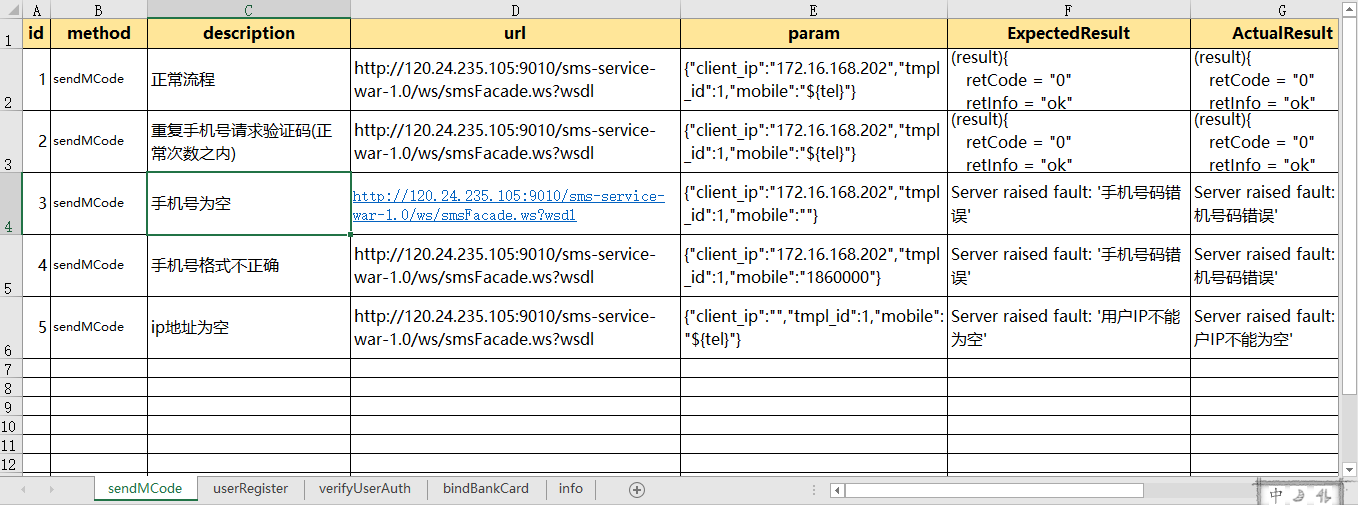1.首先我们应该先将这个模块导入

`import pandas as pd`

2.读取表单中的数据：

```sheet=pd.read_excel('test_data\\webservice_testcase.xlsx')#这个会直接默认读取到这个Excel的第一个表单
print("获取到所有的值:\n{0}".format(data))#格式化输出```

3.也可以通过指定表单名来读取数据

```sheet=pd.read_excel('test_data\\webservice_testcase.xlsx',sheet_name='userRegister')
print("获取到所有的值:\n{0}".format(data))#格式化输出```

4.通过表单索引来指定要访问的表单，0表示第一个表单,也可以采用表单名和索引的双重方式来定位表单,也可以同时定位多个表单，方式都罗列如下所示

```sheet=pd.read_excel('test_data\\webservice_testcase.xlsx',sheet_name=['sendMCode','userRegister'])#可以通过表单名同时指定多个
print("获取到所有的值:\n{0}".format(data))#格式化输出```

1.读取制定的某一行数据:

```sheet=pd.read_excel('webservice_testcase.xlsx')#这个会直接默认读取到这个Excel的第一个表单
data=sheet.ix.values#0表示第一行 这里读取数据并不包含表头
print("读取指定行的数据：\n{0}".format(data))```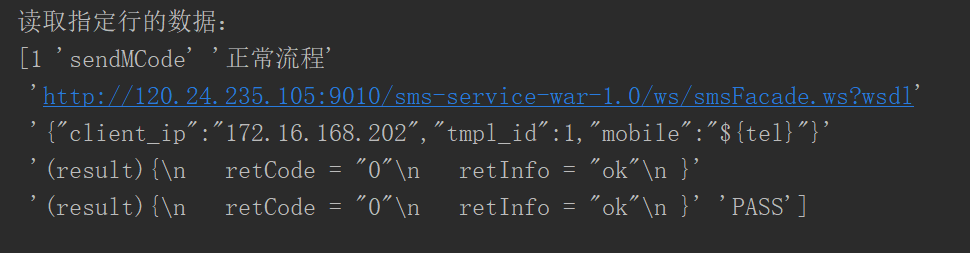2.读取指定的多行：

```sheet=pd.read_excel('test_data\\webservice_testcase.xlsx')#这个会直接默认读取到这个Excel的第一个表单
data=sheet.ix[[0,1]].values#0表示第一行 这里读取数据并不包含表头
print("读取指定行的数据：\n{0}".format(data))```3.读取指定行列的数据：

```sheet=pd.read_excel('test_data\\webservice_testcase.xlsx')#这个会直接默认读取到这个Excel的第一个表单
data=sheet.ix[0,1]#读取第一行第二列的值
print("读取指定行的数据：\n{0}".format(data))```4.读取指定的多行多列的值：

```sheet=pd.read_excel('test_data\\webservice_testcase.xlsx')
data=sheet.ix[[1,2],['method','description']].values#读取第二行第三行的method以及description列的值，这里需要嵌套列表
print("读取指定行的数据：\n{0}".format(data))```5.读取所有行指定的列的值：

```sheet=pd.read_excel('test_data\\webservice_testcase.xlsx')
data=sheet.ix[:,['method','description']].values#读取第二行第三行的method以及description列的值，这里需要嵌套列表
print("读取指定行的数据：\n{0}".format(data))```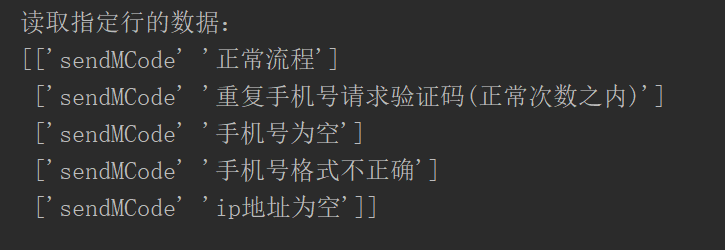6.获取行号输出：

```sheet=pd.read_excel('test_data\\webservice_testcase.xlsx')
print("输出行号列表",sheet.index.values)```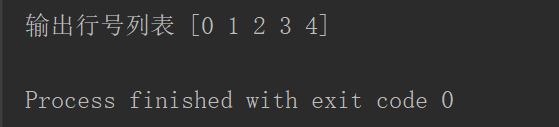7.获取列名输出：

```sheet=pd.read_excel('test_data\\webservice_testcase.xlsx')
print("输出列标题",sheet.columns.values)```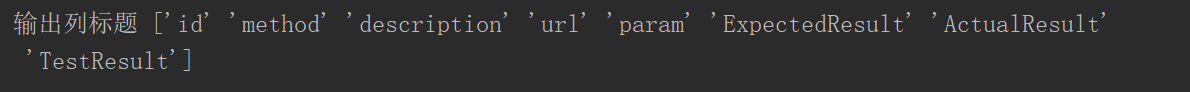8.获取指定行数的值：

```sheet=pd.read_excel('test_data\\webservice_testcase.xlsx')
print("输出值",sheet.sample(2).values)```

9.获取指定列的值

```sheet=pd.read_excel('test_data\\webservice_testcase.xlsx')
print("输出值",sheet['description'].values)```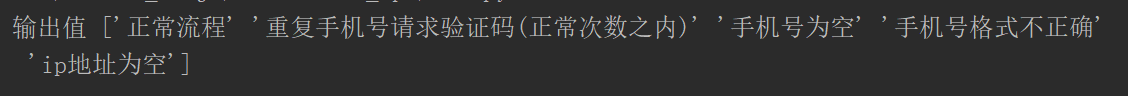```test_data=[]
for i in sheet.index.values:#获取行号的索引，并对其进行遍历：#根据i来获取每一行指定的数据 并利用to_dict转成字典
row_data=sheet.ix[i,['id','method','description','url','param','ExpectedResult']].to_dict()
test_data.append(row_data)```

```[CASECONFIG]
sheet_list={'sendMCode':'all',
'userRegister':'all',
'verifyUserAuth':'all',
'bindBankCard':[]
}```

```import configparser
cf=configparser.ConfigParser()
value=cf.get(section,option)
return value```

project_path.py代码如下：

```import os
Project_path=os.path.split(os.path.split(os.path.realpath(__file__)))
#配置文件路径
case_config_path=os.path.join(Project_path,'config','case.config')
#测试用例的路径
test_cases_path=os.path.join(Project_path,'test_data','webservice_testcase.xlsx')```

```from common import project_pathfrom common.read_config import ReadConfig as RC
import  pandas  as pd

class DoExcel:
def __init__(self,file_name):
self.file_name=file_name
def do_excel(self):
test_data=[]
for key in self.sheet_list:
if self.sheet_list[key] == 'all':  # 读取所有的用例
for i in sheet.index.values:#获取行号的索引，并对其进行遍历：
#根据i来获取每一行指定的数据 并利用to_dict转成字典
row_data=sheet.ix[i,['id','method','description','url','param','ExpectedResult']].to_dict()
test_data.append(row_data)
else:
for i in self.sheet_list[key]:#根据list中的标号去读取excel指定的用例
row_data=sheet.ix[i-1,['id','method','description','url','param','ExpectedResult']].to_dict()
test_data.append(row_data)
return  test_data
if __name__ == '__main__':
test_data=DoExcel(project_path.test_cases_path).do_excel()
print(test_data)```

```[CASECONFIG]
sheet_list={'sendMCode':[1,3,5],
'userRegister':[],
'verifyUserAuth':[],
'bindBankCard':[]
}```

```[{: 1, : , : , : , : , : },
{: 3, : , : , : , : , : },
{: 5, : , : , : , : , : }]```

Python开发开发项目